Courses

# Partial Derivatives, Gradient - MCQ Test 1

## 20 Questions MCQ Test Mock Test Series - Mechanical Engineering (ME) for GATE 2020 | Partial Derivatives, Gradient - MCQ Test 1

Description
This mock test of Partial Derivatives, Gradient - MCQ Test 1 for Mechanical Engineering helps you for every Mechanical Engineering entrance exam. This contains 20 Multiple Choice Questions for Mechanical Engineering Partial Derivatives, Gradient - MCQ Test 1 (mcq) to study with solutions a complete question bank. The solved questions answers in this Partial Derivatives, Gradient - MCQ Test 1 quiz give you a good mix of easy questions and tough questions. Mechanical Engineering students definitely take this Partial Derivatives, Gradient - MCQ Test 1 exercise for a better result in the exam. You can find other Partial Derivatives, Gradient - MCQ Test 1 extra questions, long questions & short questions for Mechanical Engineering on EduRev as well by searching above.
QUESTION: 1

### Consider the function f(x) = x2 – x – 2. The maximum value of f(x) in the closed interval [–4, 4] is

Solution: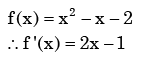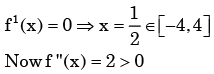∴ f (x )has minimum at x= 1 / 2 It Shows that a maximum value that will be at x = 4  or x = - 4

At x = 4, f (x )= 10

∴ At x= −4, f (x ) = 18

∴ At x= −4, f (x ) has a maximum.

QUESTION: 2

###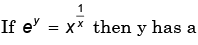Solution: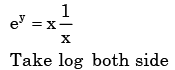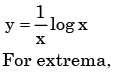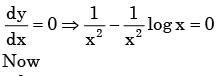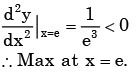QUESTION: 3

###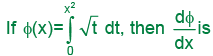Solution: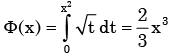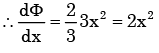QUESTION: 4

The function f(x) = x3- 6x2+ 9x+25 has

Solution: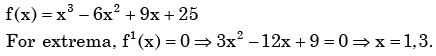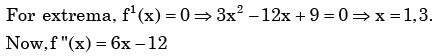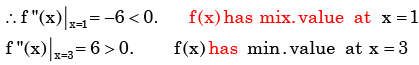QUESTION: 5

The function f(x,y) = 2x2 +2xy – y3 has

Solution: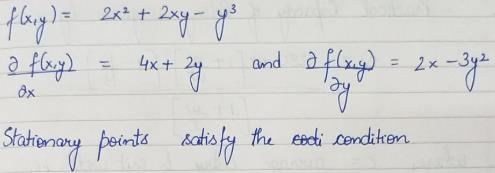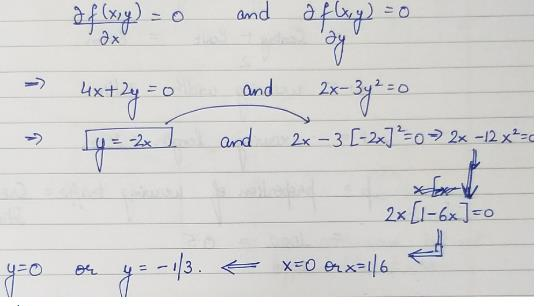QUESTION: 6

Equation of the line normal to function f(x) = (x-8)2/3+1 at P(0,5) is

Solution: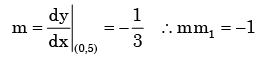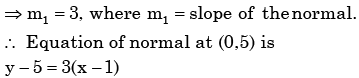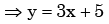QUESTION: 7

The distance between the origin and the point nearest to it on the surface z2 = 1 + xy is

Solution: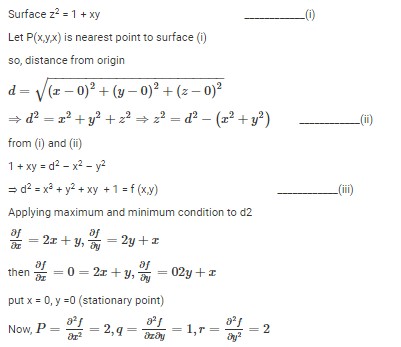or pr – q2 = 4 – 1 = 3 > 0 and r = +ve

so f(xy) is minimum at (0,0)

Hence, minimum value of d2 at (0,0)

d2 = x2 + y2 + xy + 1 = (0)2 + (0)2 + (0)(0) + 1 = 1

Then the nearest point is

z2 = 1 + xy = 1+ (0)(0) = 1

or z = 1

QUESTION: 8

Given a function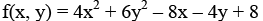The optimal value of f(x, y)

Solution: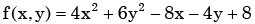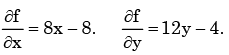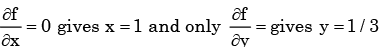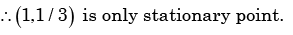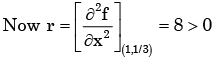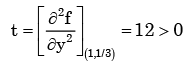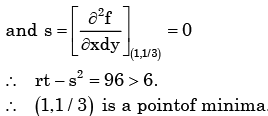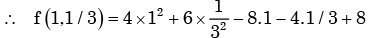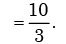QUESTION: 9

For the function f(x) = x2e-x, the maximum occurs when x is equal to

Solution: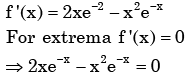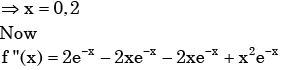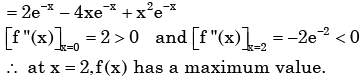QUESTION: 10

A cubic polynomial with real coefficients

Solution: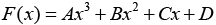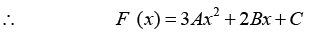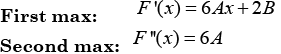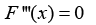So maximum two extrema and three zero crossing

QUESTION: 11

Given y = x2 + 2x + 10, the value of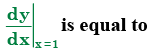Solution: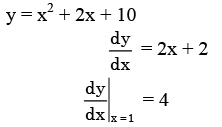QUESTION: 12

Consider the function y = x2 – 6x + 9. The maximum value of y obtained when x varies over the interval 2 to 5 is

Solution: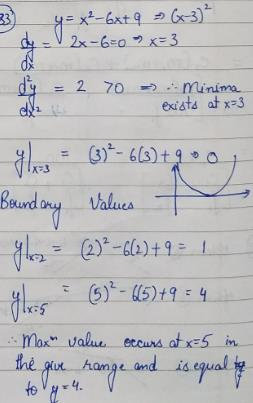QUESTION: 13

Consider the function y = x2 – 6x + 9. The maximum value of y obtained when x varies over the interval 2 to 5 is

Solution: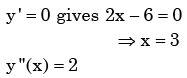QUESTION: 14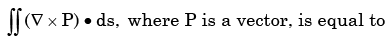Solution:
QUESTION: 15

The magnitude of the gradient of the function f = xyz3 at (1,0,2) is

Solution: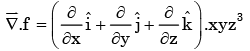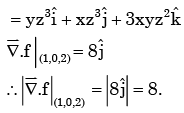QUESTION: 16

The expression curl (grad f), where f is a scalar function, is

Solution: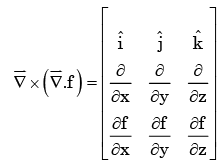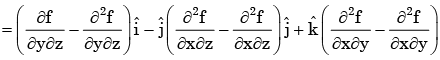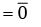QUESTION: 17

If the velocity vector in a two – dimensional flow field is given by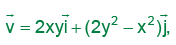the  vorticity vector, curl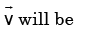Solution: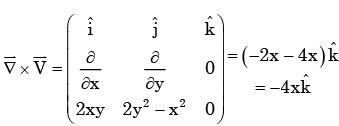QUESTION: 18

The vector field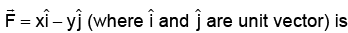Solution: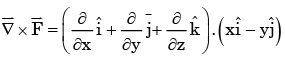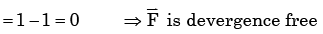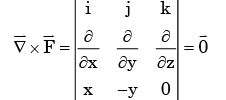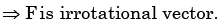QUESTION: 19

The angle between two unit-magnitude co-planar vectors P (0.866, 0.500, 0) and Q (0.259, 0.966, 0) will be

Solution: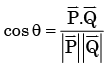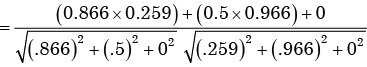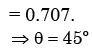QUESTION: 20

Stokes theorem connects

Solution: